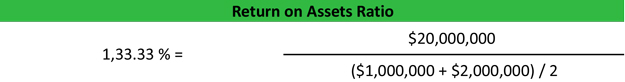# Return on Assets Ratio – ROA

The return on assets ratio, often called the return on total assets, is a profitability ratio that measures the net income produced by total assets during a period by comparing net income to the average total assets. In other words, the return on assets ratio or ROA measures how efficiently a company can manage its assets to produce profits during a period.

Since company assets’ sole purpose is to generate revenues and produce profits, this ratio helps both management and investors see how well the company can convert its investments in assets into profits. You can look at ROA as a return on investment for the company since capital assets are often the biggest investment for most companies. In this case, the company invests money into capital assets and the return is measured in profits.

In short, this ratio measures how profitable a company’s assets are.

## Formula

The return on assets ratio formula is calculated by dividing net income by average total assets.This ratio can also be represented as a product of the profit margin and the total asset turnover.

Either formula can be used to calculate the return on total assets. When using the first formula, average total assets are usually used because asset totals can vary throughout the year. Simply add the beginning and ending assets together on the balance sheet and divide by two to calculate the average assets for the year. It might be obvious, but it is important to mention that average total assets is the historical cost of the assets on the balance sheet without taking into consideration the accumulated depreciation.

The net income can be found on the income statement.

## Analysis

The return on assets ratio measures how effectively a company can earn a return on its investment in assets. In other words, ROA shows how efficiently a company can convert the money used to purchase assets into net income or profits.

Since all assets are either funded by equity or debt, some investors try to disregard the costs of acquiring the assets in the return calculation by adding back interest expense in the formula.

It only makes sense that a higher ratio is more favorable to investors because it shows that the company is more effectively managing its assets to produce greater amounts of net income. A positive ROA ratio usually indicates an upward profit trend as well. ROA is most useful for comparing companies in the same industry as different industries use assets differently. For instance, construction companies use large, expensive equipment while software companies use computers and servers.

## Example

Charlie’s Construction Company is a growing construction business that has a few contracts to build storefronts in downtown Chicago. Charlie’s balance sheet shows beginning assets of \$1,000,000 and an ending balance of \$2,000,000 of assets. During the current year, Charlie’s company had net income of \$20,000,000. Charlie’s return on assets ratio looks like this.As you can see, Charlie’s ratio is 1,333.3 percent. In other words, every dollar that Charlie invested in assets during the year produced \$13.3 of net income. Depending on the economy, this can be a healthy return rate no matter what the investment is.

Investors would have to compare Charlie’s return with other construction companies in his industry to get a true understanding of how well Charlie is managing his assets.

Contents

[i]
[i]